# Mutual Induction and Mutual Inductance with Dot Convention

## Definition of Mutual induction

Mutual induction is a phenomenon when a coil gets induced in EMF across it due to rate of change current in adjacent coil in such a way that the flux of one coil current gets linkage of another coil.

## Definition of Mutual Inductance

Mutual Inductance is the ratio between induced emf across a coil to the rate of change of current of another adjacent coil in such a way that two coils are in possibility of flux linkage.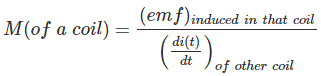## Mutual Induction

Whenever there is a time varying current in a coil, the time varying flux will link with the coil itself and will cause self induced emf across the coil. This emf is viewed as a voltage drop across the coil or inductor. But it is not practical that a coil gets linked only with its own changing flux. When a time varying current flows in another coil placed nearby the first one then the flux produced by the second coil may also link the first one. This varying flux linkage from the second coil will also induce emf across the first coil. This phenomenon is called mutual induction and the emf induced in one coil due to time varying current flowing in any other coil is called mutually induced emf. If the first coil is also connected to the time varying source, the net emf of the first coil is the resultant of self induced and mutually induced emf.

## Coefficient of Mutual Induction or Mutual Inductance

Let us consider one coil of self inductance L1 and another coil of self inductance L2. Now we will also consider that there is a low reluctance magnetic core which couples these both coils in such a way that entire flux created by one coil will link the other coil. That means there will be no leakage of flux in the system.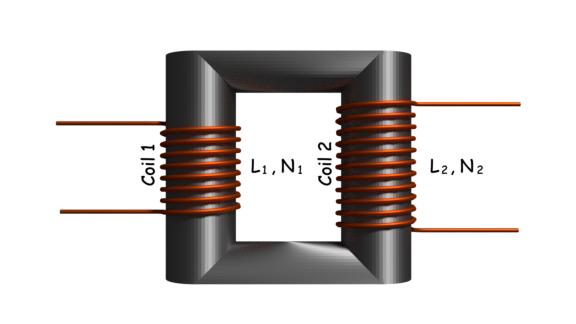Now we will apply a time varying current at coil 1 keeping the coil 2 open circuited. The voltage induced across the coil 1 will be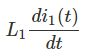Now we will keep the first coil open and apply time varying current in coil 2. Now the flux produced by coil 2 will link coil 1 through the magnetic core and as a result, the emf induced in the coil 1 will be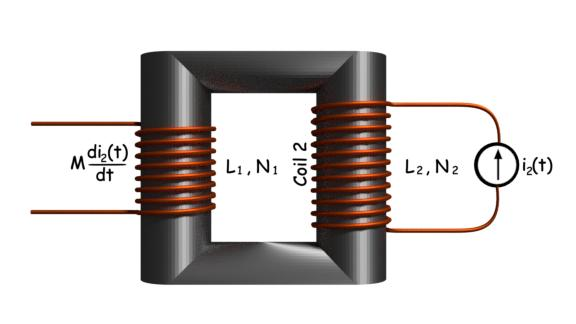Here, M is the coefficient of mutual induction or in short mutual inductance. Now without disturbing the source at coil 2, we connect a time varying current source across the coil 1. In that situation, there will be a self induced emf across the coil 1 due to its own current and also mutually induced emf across the coil 1 for the current in coil 2. So the resultant emf induced in the coil 1 is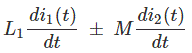Mutually induced emf may be either additive or subtractive depending on the polarity of the coil. The expression of M is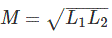This expression is justified only when entire flux created by one coil will link another coil but practically it not always possible to link entire flux of one coil to another. The value of actual mutual inductance depends on the actual amount of flux of one coil links another. Here k is a coefficient which must be multiplied with M to derive actual value of mutual inductance.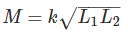## Dot Convention

As we have already told that whether the mutually induced emf would be additive or subtractive depends on the relative polarity of the mutually coupled coils. The relative polarity of two or more mutually coupled coils is denoted by dot convention. It is represented with a dot mark at either end of a coil. If at an instant, current is entering a coil through dotted end then mutually induced emf on the other coil will have the positive polarity at the dotted end of the later. It can be said in a different way that if the current is leaving a coil through the dotted end then mutually induced emf on the other coil will have the negative polarity at the dotted end of the later.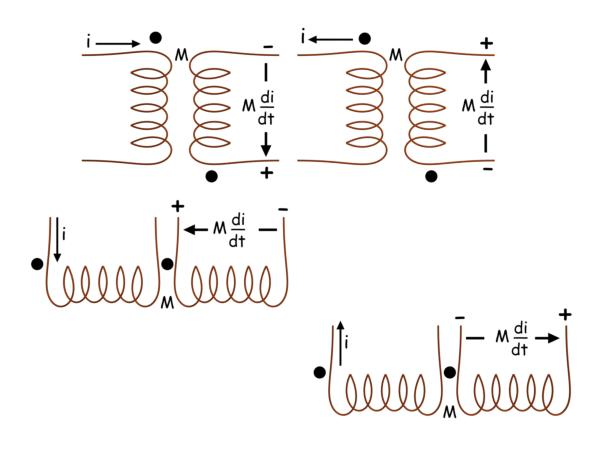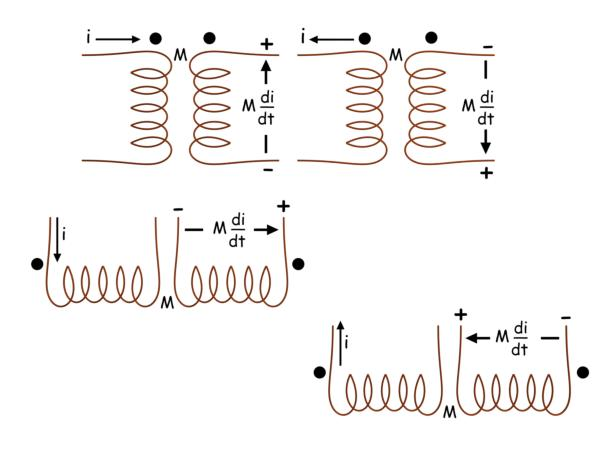Want To Learn Faster? 🎓
Get electrical articles delivered to your inbox every week.
No credit card required—it’s 100% free.